Main Content

# helixMultifilar

Creates bifilar or quadrafilar helix or conical helix antenna on circular ground plane

## Description

The `helixMultifilar` object creates a bifilar or quadrafilar helix or conical helix antenna on a circular ground plane. You can create both short-circuited and open-ended helix multifilar antennas. Bifilar and quadrafilar helix antennas are used in aerospace and defense applications.

The width of the strip is related to the diameter of an equivalent cylinder by the equation

`$w=2d=4r$`

where:

• w is the width of the strip.

• d is the diameter of an equivalent cylinder.

• r is the radius of an equivalent cylinder.

For a given cylinder radius, use the `cylinder2strip` utility function to calculate the equivalent width. The default helix antenna is end-fed. The circular ground plane is on the X-Y plane. Helix antennas are commonly used in axial mode. In this mode, the helix circumference is comparable to the operating wavelength, and the helix has maximum directivity along its axis. In normal mode, the helix radius is small compared to the operating wavelength. In this mode, the helix radiates broadside, that is, in the plane perpendicular to its axis. The basic equations for the helix are

$\begin{array}{l}x=r\mathrm{cos}\left(\theta \right)\\ y=r\mathrm{sin}\left(\theta \right)\\ z=S\theta \end{array}$

where:

• r is the radius of the helical dipole.

• θ is the winding angle.

• S is the spacing between turns.

For a given pitch angle in degrees, use the `helixpitch2spacing` utility function to calculate the spacing between the turns in meters.## Creation

### Syntax

``ant = helixMultifilar``
``ant = helixMultifilar(Name,Value)``

### Description

example

````ant = helixMultifilar` creates a bifilar or quadrafilar helix or conical helix antenna operating in the axial mode. The default multifilar helical antenna is end-fed and has a circular ground plane on the X-Y plane. The default operating frequency is around 2 GHz.```

example

````ant = helixMultifilar(Name,Value)` sets properties using one or more name-value pairs. For example, `ant = helixMultifilar('Radius',28e-03)` creates a multifilar helix with turns of radius 28e-03 m.```

## Properties

expand all

Number of helical elements, specified as `4` or `2`. Specify two elements to create a bifilar helix antenna, and four elements to create a quadrafilar helix antenna.

Example: `'NumArms',2`

Example: `ant.NumArms = 2`

Data Types: `double`

Radius of the turns, specified as a positive scalar integer in meters or a two element vector with each element unit in meters. In the two-element vector, the first element specifies the bottom radius and the second element specifies the top radius of the conical helix antenna.

Example: `'Radius',28e-03`

Example: `ant.Radius = 28e-03`

Data Types: `double`

Width of the strip, specified as a positive scalar integer in meters.

Example: `'Width',0.2`

Example: `ant.Width = 0.2`

Data Types: `double`

Number of turns, specified as a scalar integer.

Example: `'Turns',4`

Example: `ant.Turns = 4`

Data Types: `double`

Spacing between the turns, specified as a positive scalar integer in meters.

Example: `'Spacing',7.5e-2`

Example: `ant.Spacing = 7.5e-2`

Data Types: `double`

Status of helix ends, specified as `0` or `1`. By default, the `helixMultifilar` is an open circuit. Setting the property to `1` makes the helix antenna short circuit.

Example: `'ShortEnds',1`

Example: `ant.ShortEnds = 1`

Data Types: `double`

Direction of the helix turns (windings), specified as `'CW'` for clockwise or `'CCW'` for counter-clockwise.

Example: `'WindingDirection','CW'`

Example: `ant.WindingDirection = 'CW'`

Data Types: `char` | `string`

Height of the feeding stub from the ground plane, specified as a positive scalar integer in meters.

Example: `'FeedStubHeight',7.5e-2`

Example: `ant.FeedStubHeight = 7.5e-2`

Data Types: `double`

Ground plane radius, specified as a positive scalar integer in meters. By default, the ground plane is on the X-Y plane and is symmetrical about the origin.

Setting this value to `Inf` uses the infinite ground plane technique for antenna analysis.

Example: `'GroundPlaneRadius',2.05`

Example: `ant.GroundPlaneRadius = 7.5e-2`

Data Types: `double`

Excitation voltage applied to individual antenna feeds, specified as a scalar integer or vector integers. A scalar value applies the same voltage to all feeds.

Example: `'FeedVoltage',[1 2]`

Example: `ant.FeedVoltage = [1 2]`

Data Types: `double`

Excitation voltage phase applied to individual antenna feeds, specified as a scalar integer or vector integers. A scalar value applies the same voltage phase to all feeds.

Example: `'FeedPhase',[0 45]`

Example: `ant.FeedPhase = [0 45]`

Data Types: `double`

Type of the metal used as a conductor, specified as a metal material object. You can choose any metal from the `MetalCatalog` or specify a metal of your choice. For more information, see `metal`. For more information on metal conductor meshing, see Meshing.

Example: ```m = metal('Copper'); 'Conductor',m```

Example: ```m = metal('Copper'); ant.Conductor = m```

Lumped elements added to the antenna feed, specified as a lumped element object handle. You can add a load anywhere on the surface of the antenna. By default, the load is at the origin. For more information, see `lumpedElement`.

Example: `'Load',lumpedelement`. `lumpedelement` is the object handle for the load created using `lumpedElement`.

Example: ```ant.Load = lumpedElement('Impedance',75)```

Data Types: `double`

Tilt angle of the antenna, specified as a scalar or vector with each element unit in degrees. For more information, see Rotate Antennas and Arrays.

Example: `'Tilt',90`

Example: `ant.Tilt = 90`

Example: `'Tilt',[90 90]`,`'TiltAxis',[0 1 0;0 1 1]` tilts the antenna at 90 degrees about the two axes defined by the vectors.

Note

The `wireStack` antenna object only accepts the dot method to change its properties.

Data Types: `double`

Tilt axis of the antenna, specified as:

• Three-element vector of Cartesian coordinates in meters. In this case, each coordinate in the vector starts at the origin and lies along the specified points on the X-, Y-, and Z-axes.

• Two points in space, each specified as three-element vectors of Cartesian coordinates. In this case, the antenna rotates around the line joining the two points in space.

• A string input describing simple rotations around one of the principal axes, 'X', 'Y', or 'Z'.

For more information, see Rotate Antennas and Arrays.

Example: `'TiltAxis',[0 1 0]`

Example: `'TiltAxis',[0 0 0;0 1 0]`

Example: `ant.TiltAxis = 'Z'`

Note

The `wireStack` antenna object only accepts the dot method to change its properties.

Data Types: `double`

## Object Functions

 `show` Display antenna or array structure; display shape as filled patch `axialRatio` Axial ratio of antenna `beamwidth` Beamwidth of antenna `charge` Charge distribution on metal or dielectric antenna or array surface `current` Current distribution on metal or dielectric antenna or array surface `design` Design prototype antenna or arrays for resonance at specified frequency `efficiency` Radiation efficiency of antenna `EHfields` Electric and magnetic fields of antennas; Embedded electric and magnetic fields of antenna element in arrays `impedance` Input impedance of antenna; scan impedance of array `mesh` Mesh properties of metal or dielectric antenna or array structure `meshconfig` Change mesh mode of antenna structure `optimize` Optimize antenna or array using SADEA optimizer `pattern` Radiation pattern and phase of antenna or array; Embedded pattern of antenna element in array `patternAzimuth` Azimuth pattern of antenna or array `patternElevation` Elevation pattern of antenna or array `returnLoss` Return loss of antenna; scan return loss of array `sparameters` S-parameter object `vswr` Voltage standing wave ratio of antenna

## Examples

collapse all

Create and view a Quadrafilar helix antenna.

`ant = helixMultifilar`
```ant = helixMultifilar with properties: NumArms: 4 Radius: 0.0220 Width: 1.0000e-03 Turns: 3 Spacing: 0.0350 ShortEnds: 0 WindingDirection: 'CCW' FeedStubHeight: 1.0000e-03 GroundPlaneRadius: 0.0750 FeedVoltage: 1 FeedPhase: 0 Conductor: [1x1 metal] Tilt: 0 TiltAxis: [1 0 0] Load: [1x1 lumpedElement] ```
`show(ant)`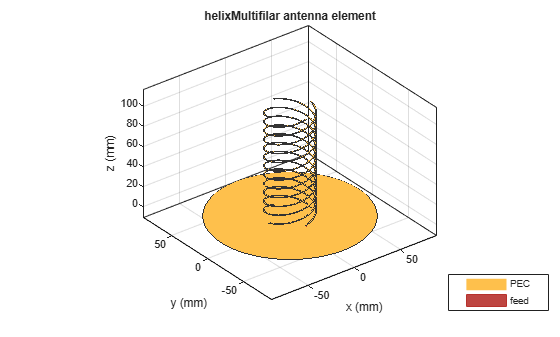Create and view a bifilar helix antenna.

`ant=helixMultifilar('NumArms',2)`
```ant = helixMultifilar with properties: NumArms: 2 Radius: 0.0220 Width: 1.0000e-03 Turns: 3 Spacing: 0.0350 ShortEnds: 0 WindingDirection: 'CCW' FeedStubHeight: 1.0000e-03 GroundPlaneRadius: 0.0750 FeedVoltage: 1 FeedPhase: 0 Conductor: [1x1 metal] Tilt: 0 TiltAxis: [1 0 0] Load: [1x1 lumpedElement] ```
`show(ant)`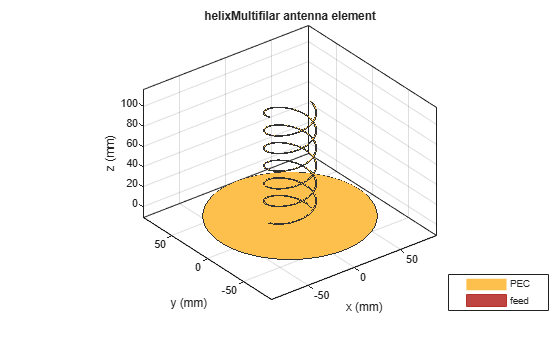Create and view a conical multifilar helix antenna of radii, 0.0220 m and 0.00800 m respectively.

`ant = helixMultifilar('Radius',[0.0080,0.0220],'ShortEnds',1)`
```ant = helixMultifilar with properties: NumArms: 4 Radius: [0.0080 0.0220] Width: 1.0000e-03 Turns: 3 Spacing: 0.0350 ShortEnds: 1 WindingDirection: 'CCW' FeedStubHeight: 1.0000e-03 GroundPlaneRadius: 0.0750 FeedVoltage: 1 FeedPhase: 0 Conductor: [1x1 metal] Tilt: 0 TiltAxis: [1 0 0] Load: [1x1 lumpedElement] ```
`show(ant)`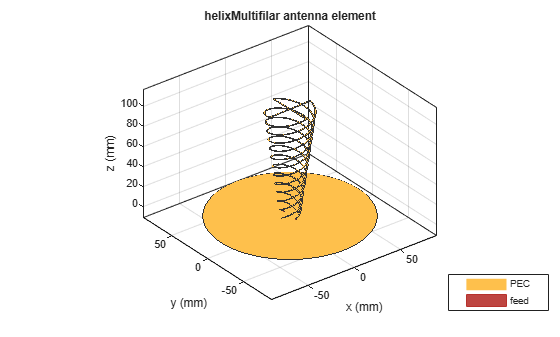Plot the pattern of the antenna at 3 GHz.

`pattern(ant,3e9)`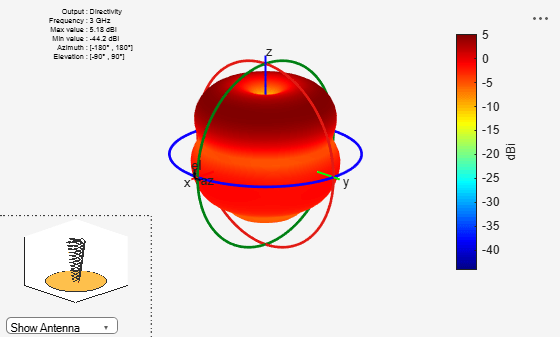Overlay the antenna on the pattern.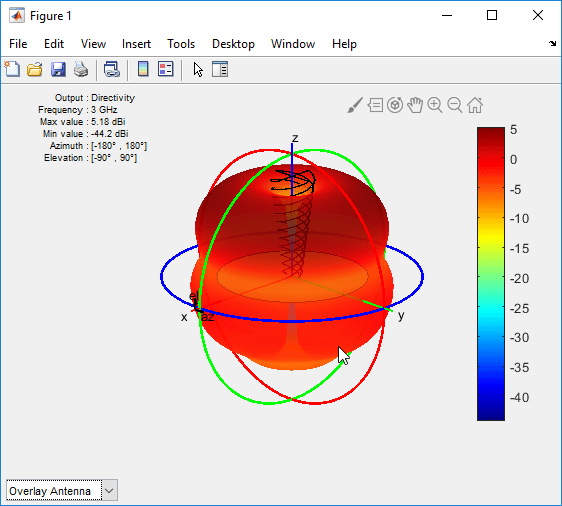## See Also

Introduced in R2018b

## Support

#### Hybrid Beamforming for Massive MIMO Phased Array Systems

Download the white paper